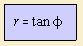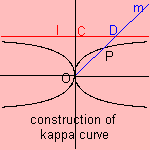# kappa curve

## quarticThis curve resembling the Greek character kappa (k) (in fact its form is more a character 'x') can be defined as follows: let the line l be parallel to the x-axis, intersecting the y-axis in point C. Consider now lines m through O that meet line l in point D. Then the kappa curve is defined as the collection of points P, for which OP = CD. Another name for the curve is Gutschoven's curve, named after the first one who studied the curve, being G. van Gutschoven (in 1662). The curve has also been studied by Newton and, later, by Johann Bernoulli and de Sluze (1862).The curve is the radial of the tractrix. In Cartesian coordinates the curve can be written as: (x2 + y2) y2 = x2 The curve is a special case of a nodal curve.